#### You may also like### Reflect Again

Follow hints to investigate the matrix which gives a reflection of the plane in the line y=tanx. Show that the combination of two reflections in intersecting lines is a rotation.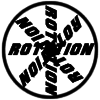### Rots and Refs

Follow hints using a little coordinate geometry, plane geometry and trig to see how matrices are used to work on transformations of the plane.### Sine Problem

In this 'mesh' of sine graphs, one of the graphs is the graph of the sine function. Find the equations of the other graphs to reproduce the pattern.

# Squaring the Rectangle

##### Age 14 to 18 Challenge Level:

Minh from BVIS in Vietnam sent an image of the pieces from the interactivity made into a square. This in Minh's image: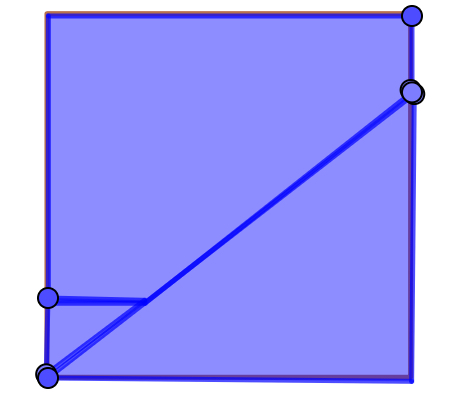(which comes from this rectangle)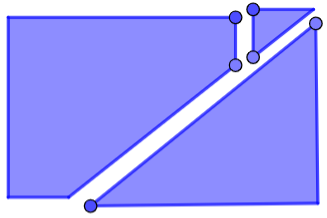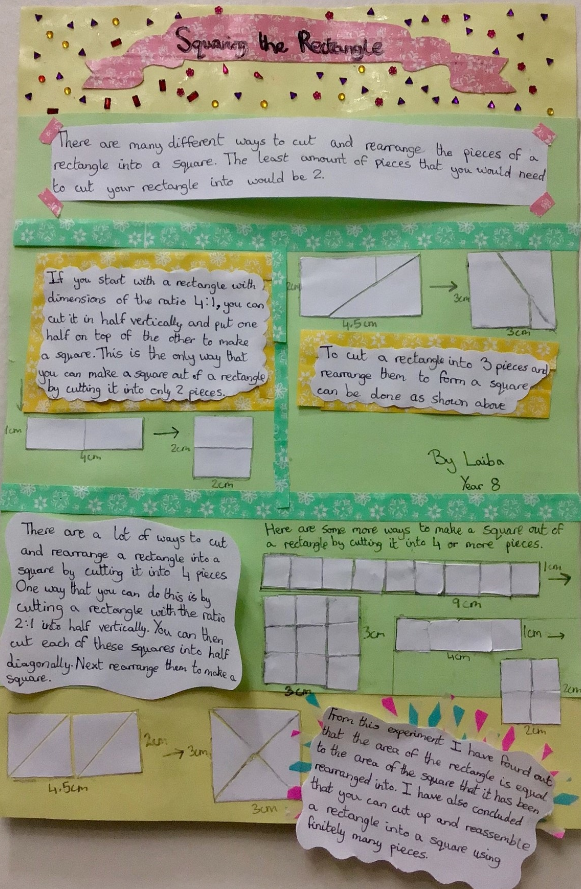Laiba from Doha College in Qatar made a beautiful poster with details of several different approaches.

Click here to see a larger version of Laiba's poster.

Minee & Pin M & Gor-Bua from Bangkok Patana School in Thailand and Ana also used a rectangle with dimensions in the ratio 2:1. Ana started with this rectangle:

The rectangle has length to width ratio $2:1.$ As an example the width is $1$ cm and the length is $2$ cm.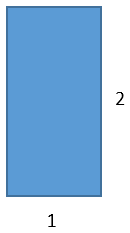The area of the rectangle and square must be equal. In this case it must be $2$ cm$^2$. The square has equal length sides and therefore the sides will have length $\sqrt2$ cm.

If the second rectangle drawn is cut into four pieces, across the diagonal of each $1\times 1$ square which is shown right, then the length of diagonals will be $1^2+1^2=a^2$ and thus $a=\sqrt2.$

The yellow triangle’s hypotenuse has length $\sqrt2,$ which is the [side] length of the square which will be constructed. A total of four yellow triangles can be cut out from the original rectangle and rearranged to create a square like this: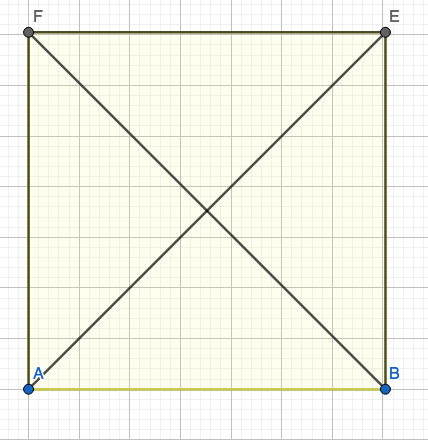The triangles had length $\sqrt2$ and two sides with length $1.$ The diagonal of the square is $2,$ which is equal to $2$ times $1$ cm, the width and half of the length of the previous rectangle.

This can be done with any triangle with ratio $2:1.$ Instead of the sides being $2$ and $1$ they can be called $a$ and $2a.$ The area of the rectangle and square would then be $2a^2.$ The sides of the square are $\sqrt2a.$

This formula can be [verified] with our example of sides $2$ and $1.$ If $1$ is $a$ then the sides of the square is $\sqrt2\ast1=\sqrt2$

Minee & Pin M & Gor-Bua proved that this formula works:
Because the lengths are "$a$" and "$2a$", to find the area of the rectangle you'll have to do $a \times 2a = 2a^2$
Because we are not cutting out any of the rectangle's area, the area of the square must be $2a^2$
To find the side lengths of the square you'll have to do $\sqrt{(2a^2)}$ because you have to do $x\times x$ to find the area ($x=\sqrt{(2a^2)}$).

David from LAE Tottenham in the UK generalised Min's method so that it will work for any rectangle:
Take any rectangle of size $a\times b$: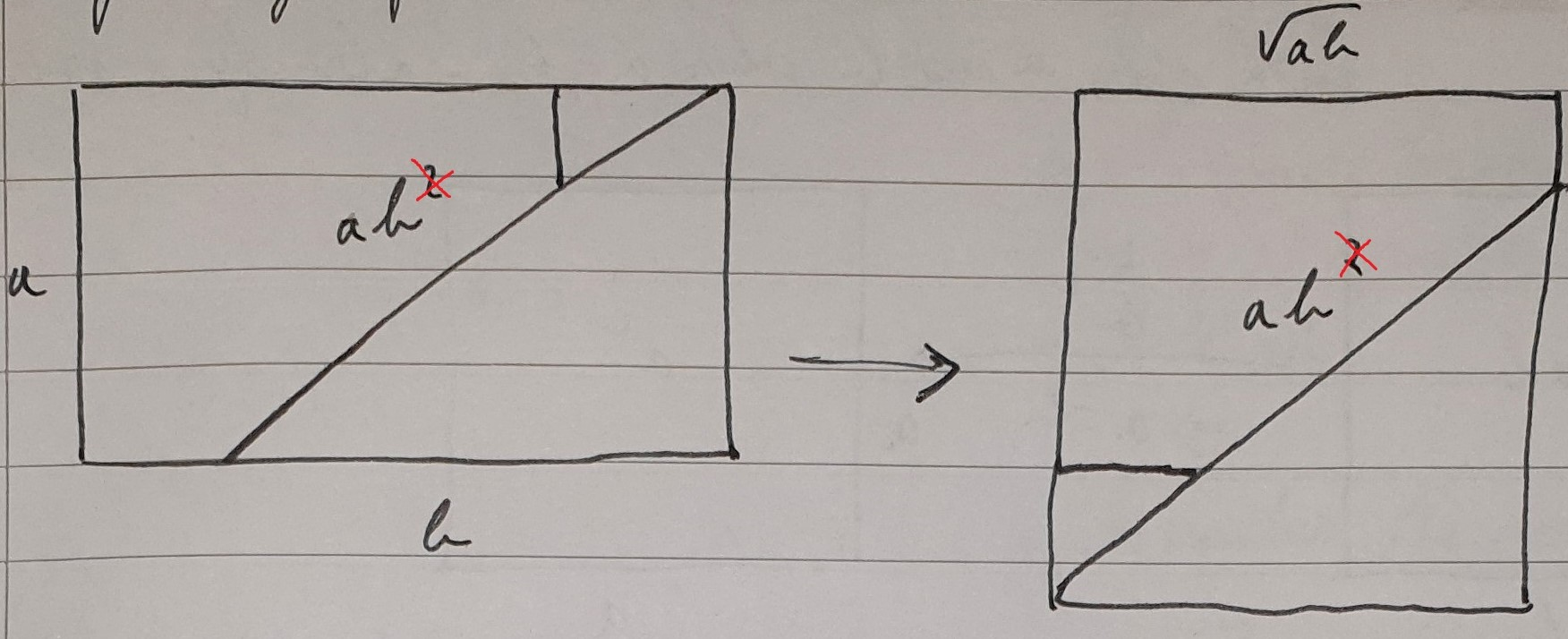height $=a$
width $=b$ where $a$ and $b$ are positive and $\left \lfloor \frac {b}{a} \right \rfloor= 1$
Where $\left \lfloor x \right \rfloor$ means the highest whole number $n$ which is less than or equal to $x$ (i.e. $x$ rounded down to the nearest whole number).We cannot use this division of the rectangles when $\left \lfloor \frac {b}{a} \right \rfloor > 1,$ as if we do the same, we do not get a square:

This process could be repeated $N$ times, and eventually $\left \lfloor \frac ba \right \rfloor =1,$ which means that it can be transformed into a square.

It should be done as follows:

1. Start with the rectangle of size $a \times b$ where $\left \lfloor \frac ba \right \rfloor > 1.$2. Find the highest square number $n^2$ fitting into $b$ such that $\left \lfloor \frac an \right \rfloor =1$ or $\left \lfloor \frac na \right \rfloor =1.$ Divide $b$ into $n^2$ even lengths (Note $\frac b {n^2}$ doesn't have to be an integer), forming new rectangles ($n=2$ shown in example)3. Perform the previous method on each rectangle, to get $n^2$ squares of area $\frac{ab}{n^2}:$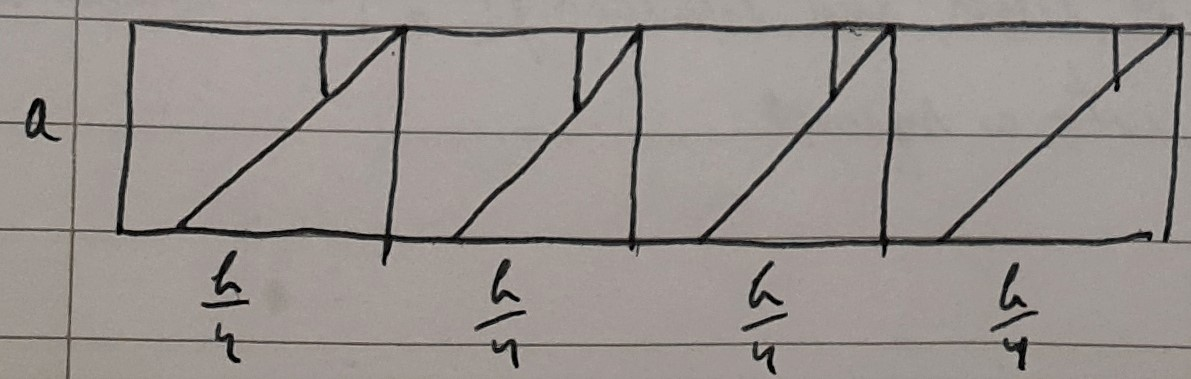4. Rearrange the pieces to form squares: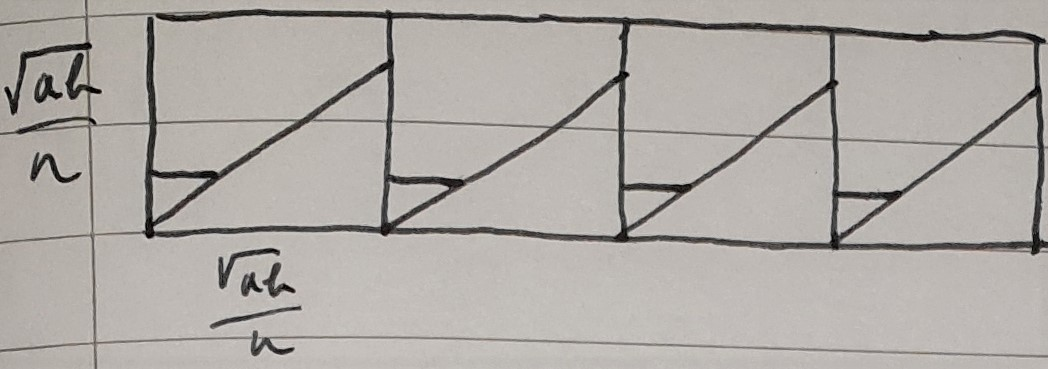each square has area $\frac{ab}{n^2},$ but there are $n^2$ squares, so the total area is still $ab^2$

5. Since there are $n^2$ squares, and $n^2$ is a square number, the rectangle can be rearranged into a square of area $ab$.